# How to make a Histogram

How to use ggplot

tutorial
Author

Miriam Heiss

Published

July 30, 2022

A Histogram is another kind of graph that you can make with `ggplot()`. You start the same way as a scatterplot, but you have a different `geom`. You only provide an x in `aes` because the Histogram builds the y for you. Let’s start!

## Libraries

Here, I’m loading my libraries. The package tidyverse has eight packages in it, including ggplot. The package primer.data has more datasets than the ones built into R.

Code
``````library(tidyverse)
library(primer.data)``````

## Choosing the Data

Here, we are going to run the code `data()`. This will show us a list of datasets we can use.

Code
``data()``

Let’s use the data nobel. If you want to see the dataset, type `glimpse(nobel)` into your console

## Starting the Histogram

It’s time to start plotting! Type `ggplot()`. That will be the start of your graph. If you run it now, it will be blank, because we don’t have any data yet!

Code
``ggplot()``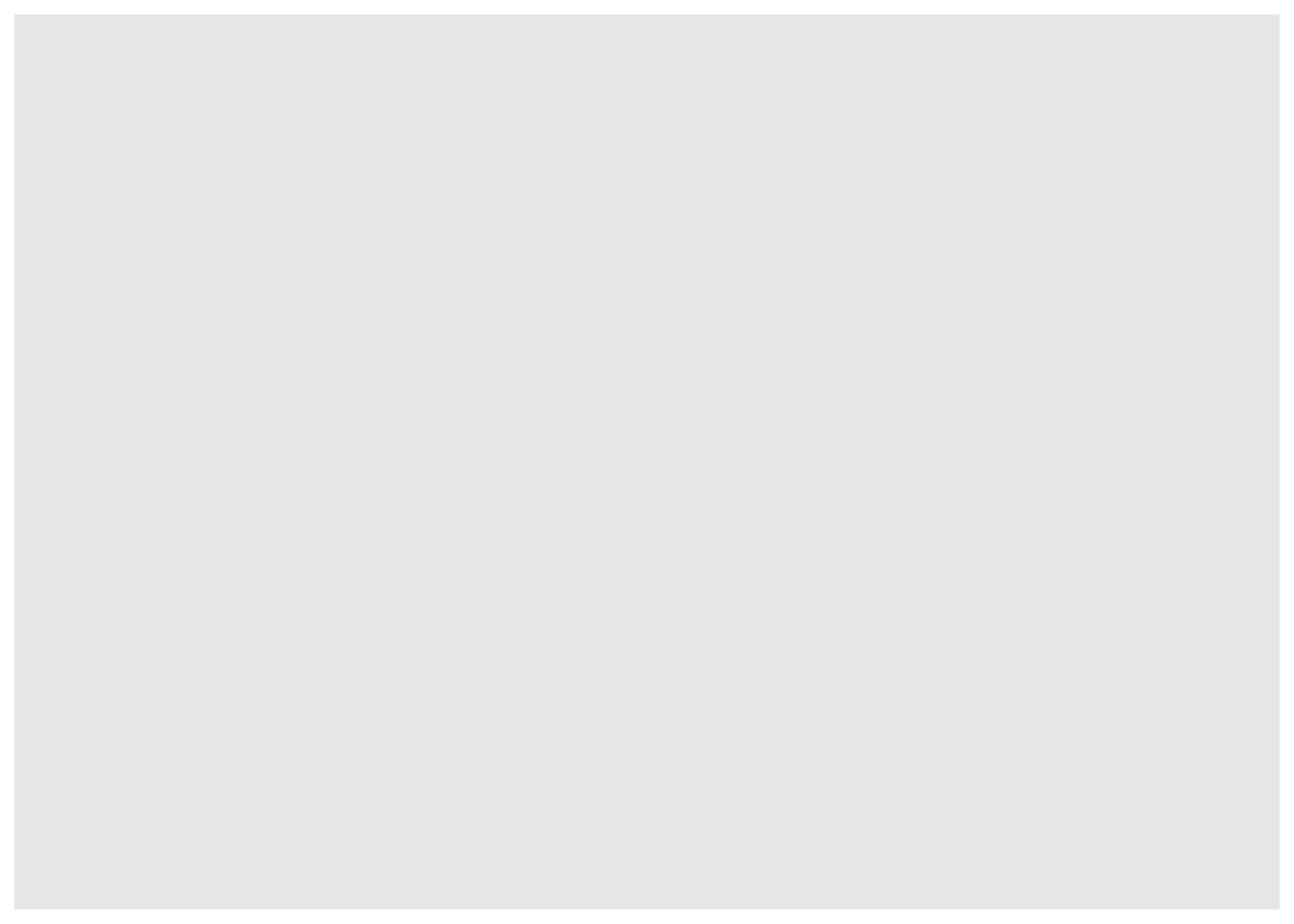Now we add the data to it inside of `ggplot()`. Set data equal to nobel.

Code
``ggplot(data = nobel)``It should still be blank, because we have not added an `x` or a `geom`. Let’s set x = year, inside of `mapping = aes()`.

Code
``````ggplot(data = nobel,
mapping = aes(x = year))``````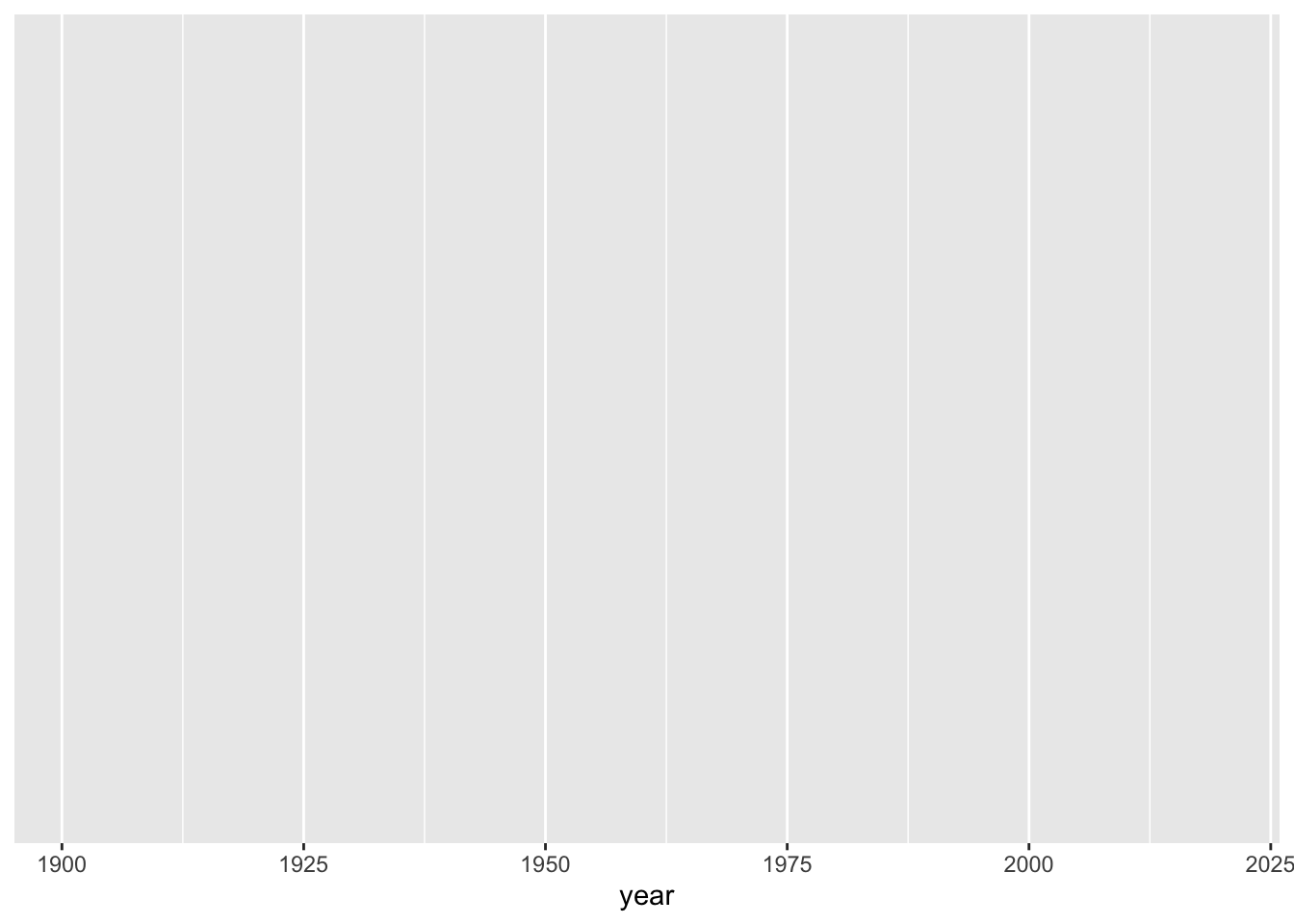Great! Now we have an empty graph, instead of a blank canvas!

## The Geom

Now, we need to add a `geom`. Add another layer with ‘+’. Make the `geom` a Histogram.

Code
``````ggplot(data = nobel,
mapping = aes(x = year)) +
geom_histogram()``````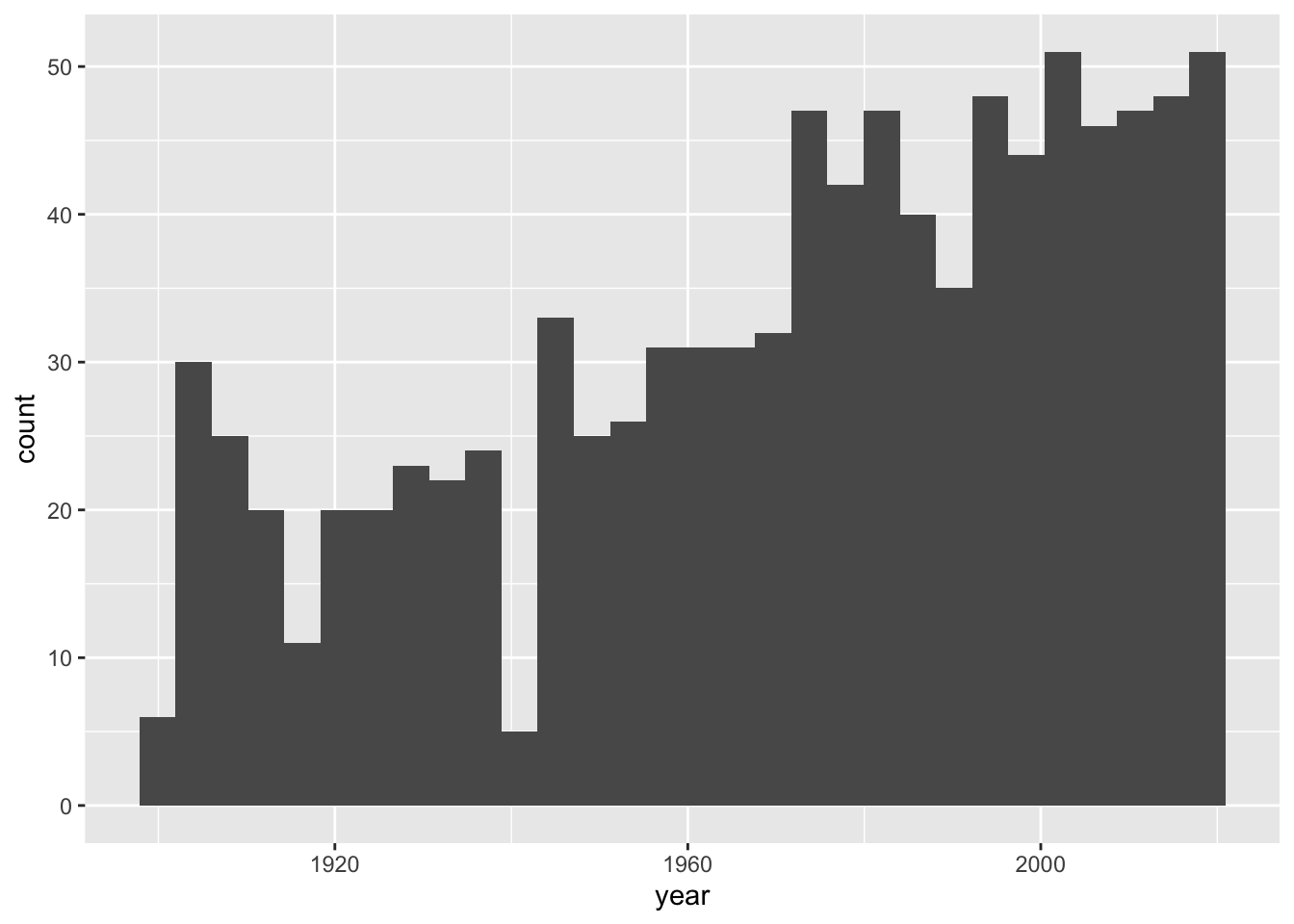Now, we are going to add color!

## Borders and Fill

There are 2 different types of color in Histograms. Fill and Color. Color is an argument within `geom_histogram()`, and fill is an argument in `aes` We are going to change fill to field, and color to white

Code
``````ggplot(data = nobel,
mapping = aes(x = year,
fill = field)) +
geom_histogram(color = "white")``````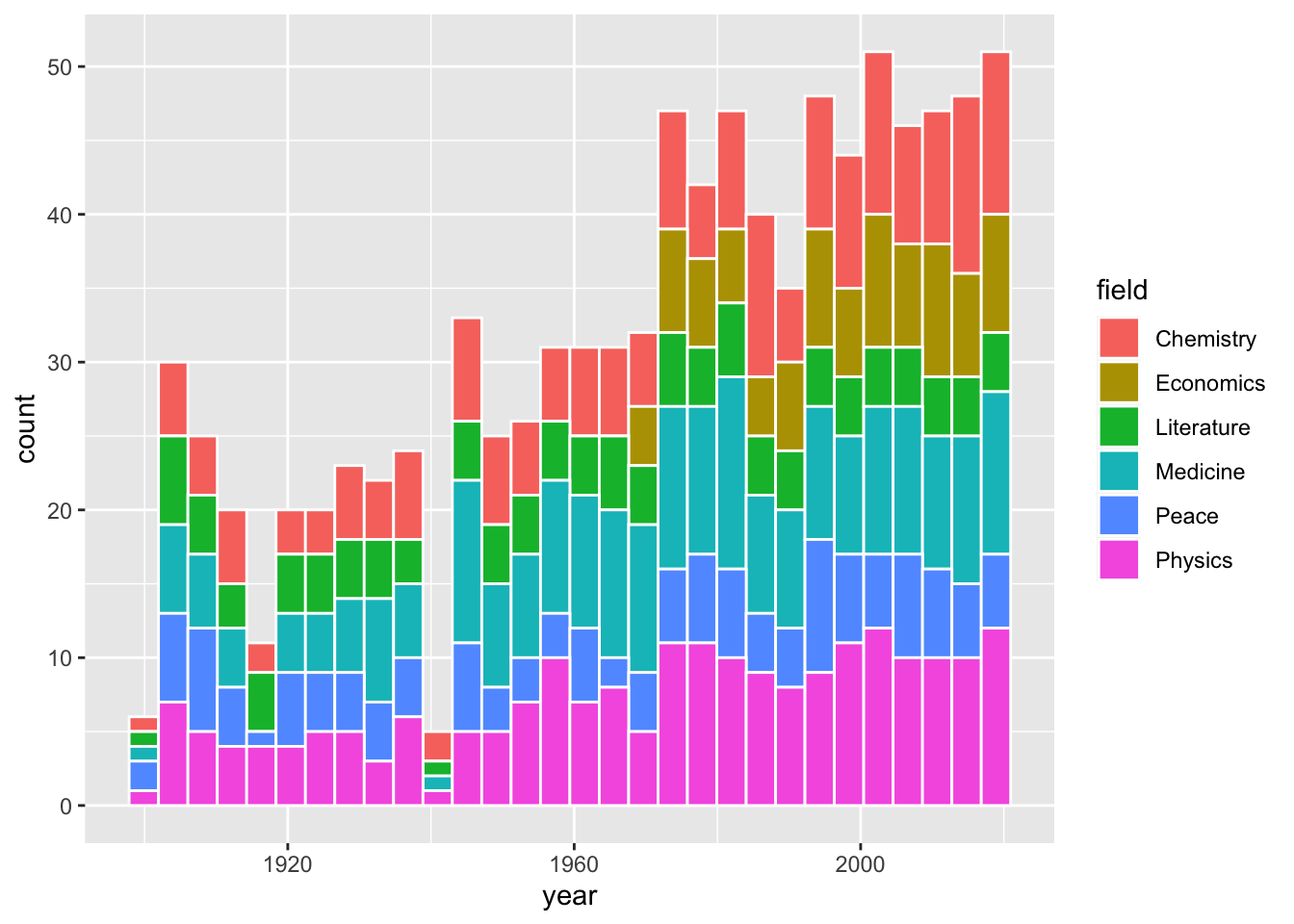That looks super nice! Now we can see the separate sections of each bin in the Histogram.

### Changing the Fill Colors (1)

Now what if we want to change the fill colors of each of the sections? That is going to be a separate argument, we will put it before the `geom` called `scale_fill_manual`. We need to choose 6 distinct colors to put in the argument. The `(values = c())` makes it a list. Let’s just use the rainbow for our colors, as all of them are built in to R. Remember to add a ‘+’ after `"purple"))` or there will just be an empty graph.

Code
``````ggplot(data = nobel,
mapping = aes(x = year,
fill = field)) +
scale_fill_manual(values = c("red",
"orange",
"yellow",
"green",
"blue",
"purple")) +
geom_histogram(color = "white")``````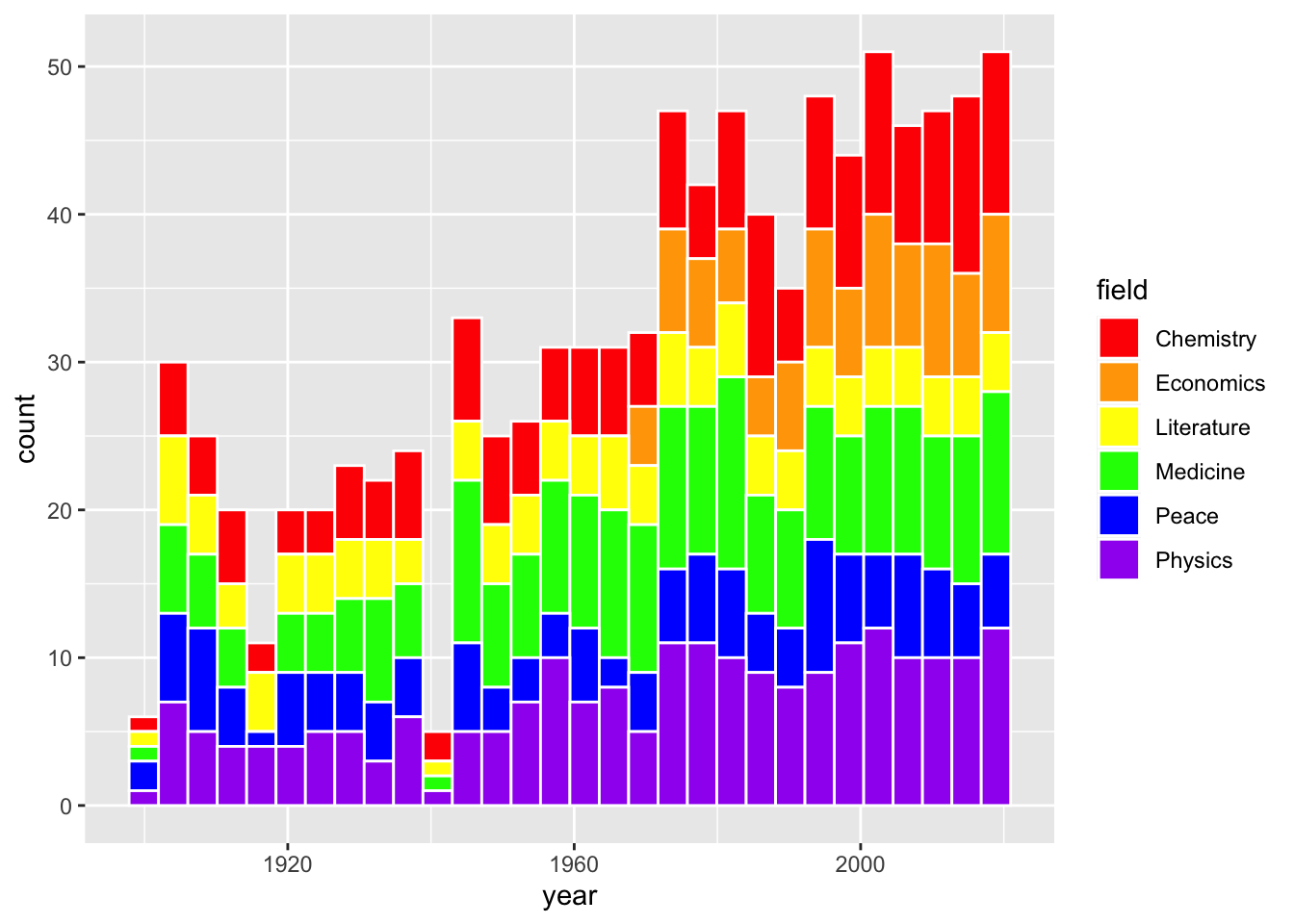That looks great! Those colors are really bright, though. What if we want custom colors?

### Changing the Fill Colors (2)

Now, we know that we can use the built in colors, but we can also get HEX colors! I found 6 different HEX colors with an online color picker.

Code
``````ggplot(data = nobel,
mapping = aes(x = year,
fill = field)) +
scale_fill_manual(values = c("#f73c39",
"#f79b39",
"#f7ee39",
"#228c14",
"#1e80c7",
"#7c148c")) +
geom_histogram(color = "white")``````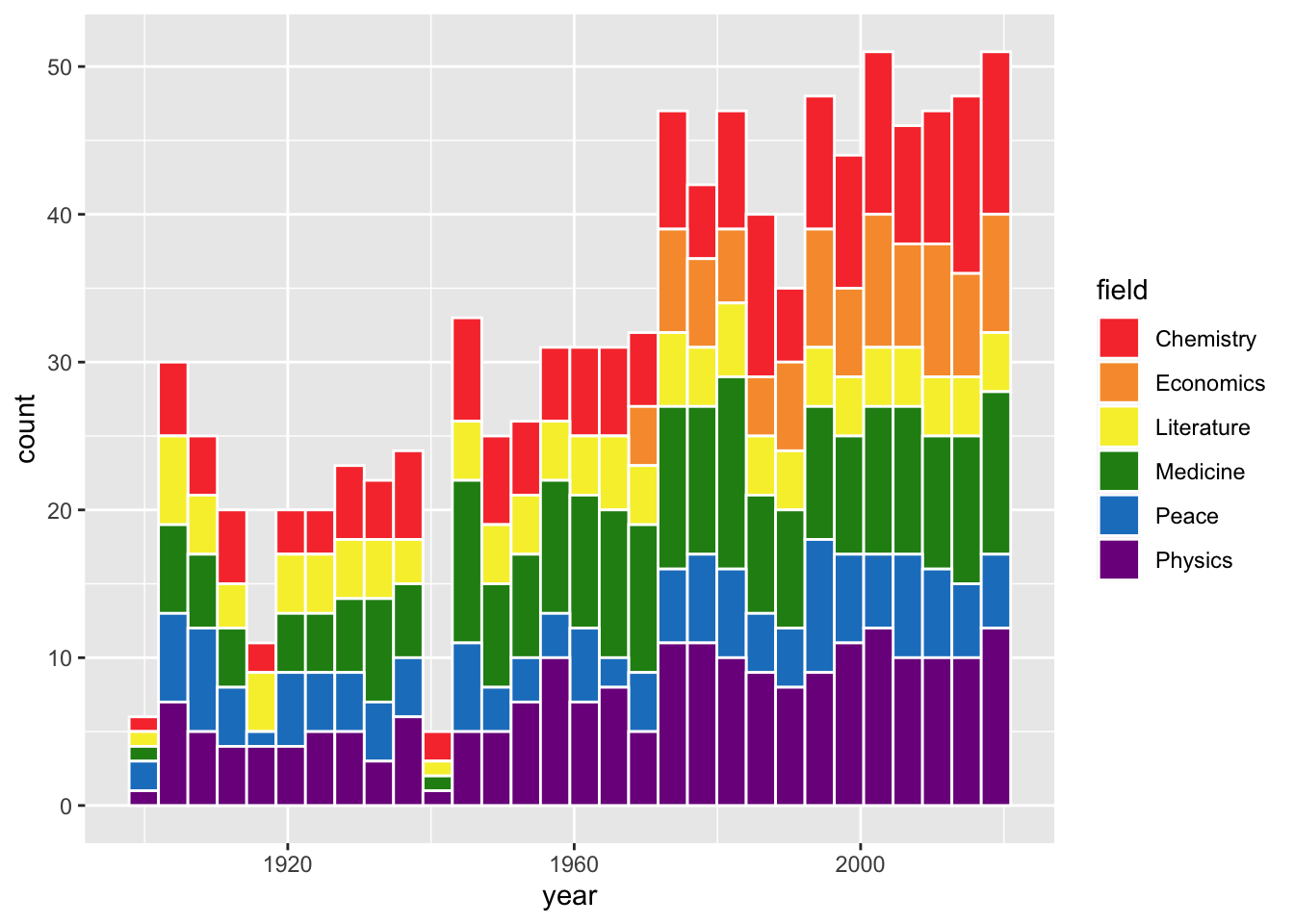### Changing the Fill Colors (3)

Here is one last way to change the fill colors in a Histogram. We will need to load another Library at the top, called MetBrewer. This has many color palettes from art at the MET. Let’s load it in!

Code
``library(MetBrewer)``

Now let’s add it to our graph.

Code
``````ggplot(data = nobel,
mapping = aes(x = year,
fill = field)) +
scale_fill_manual(values = met.brewer("Signac", 6)) +
geom_histogram(color = "white")``````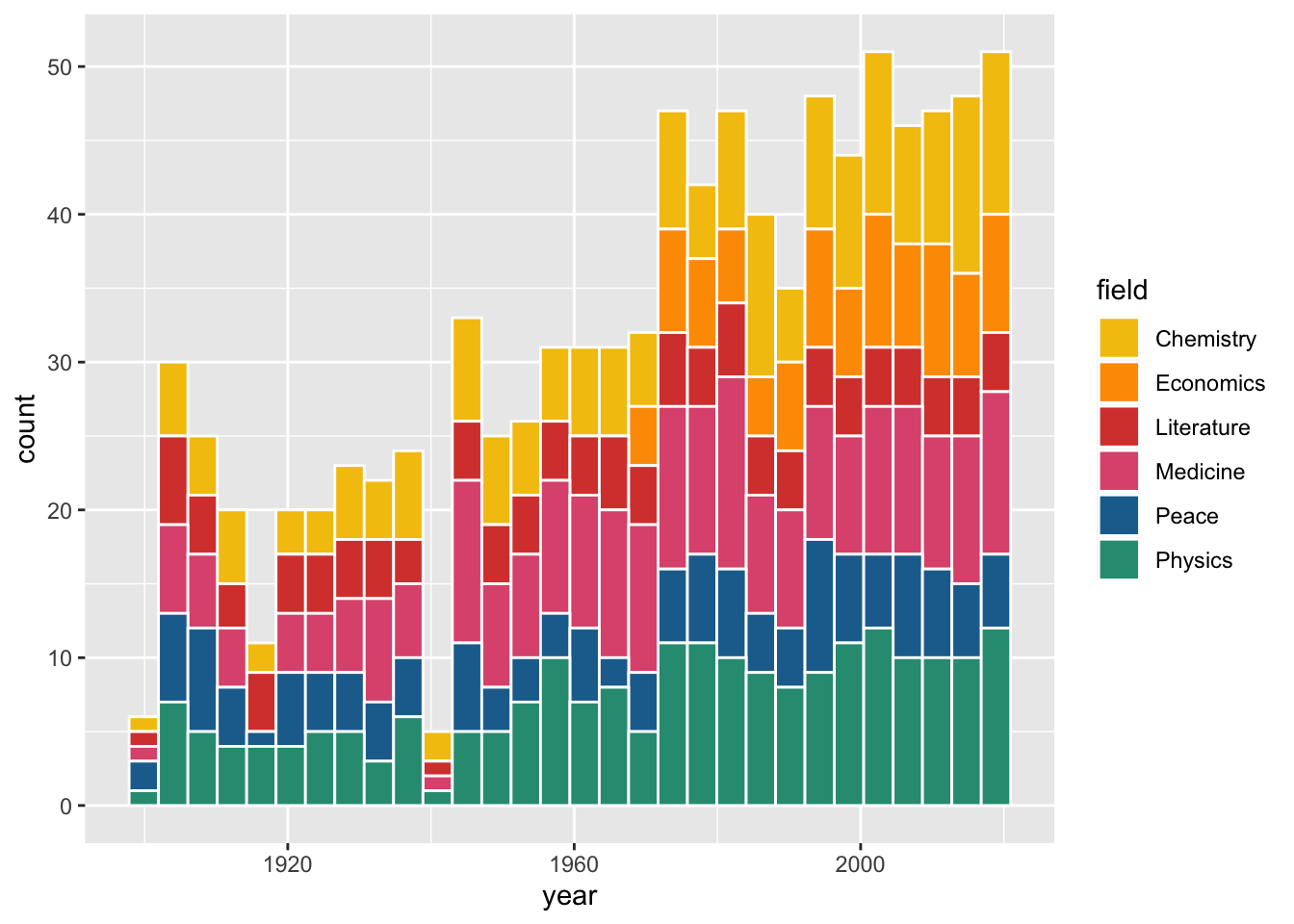I used “Signac”, but there are a lot more options. Just make sure you choose one with enough colors for your legend.

## Labeling

This graph looks nice so far:But what is the title, what are the axes called, and why is the legend lowercase?

This is where the function `labs()` comes in. `labs()` is short for labels, and it allows you to add a title, subtitle, x axis name, y axis name, and caption. Let’s add some labels.

Code
``````ggplot(data = nobel,
mapping = aes(x = year,
fill = field)) +
scale_fill_manual(values = met.brewer("Signac", 6)) +
geom_histogram(color = "white") +
labs(title = "Nobel prize laureate numbers have gone up in past 50 years",
subtitle = "Physics, Medicine, and Chemistry have largest numbers of laureates",
x = "Year",
y = "Number of laureates")``````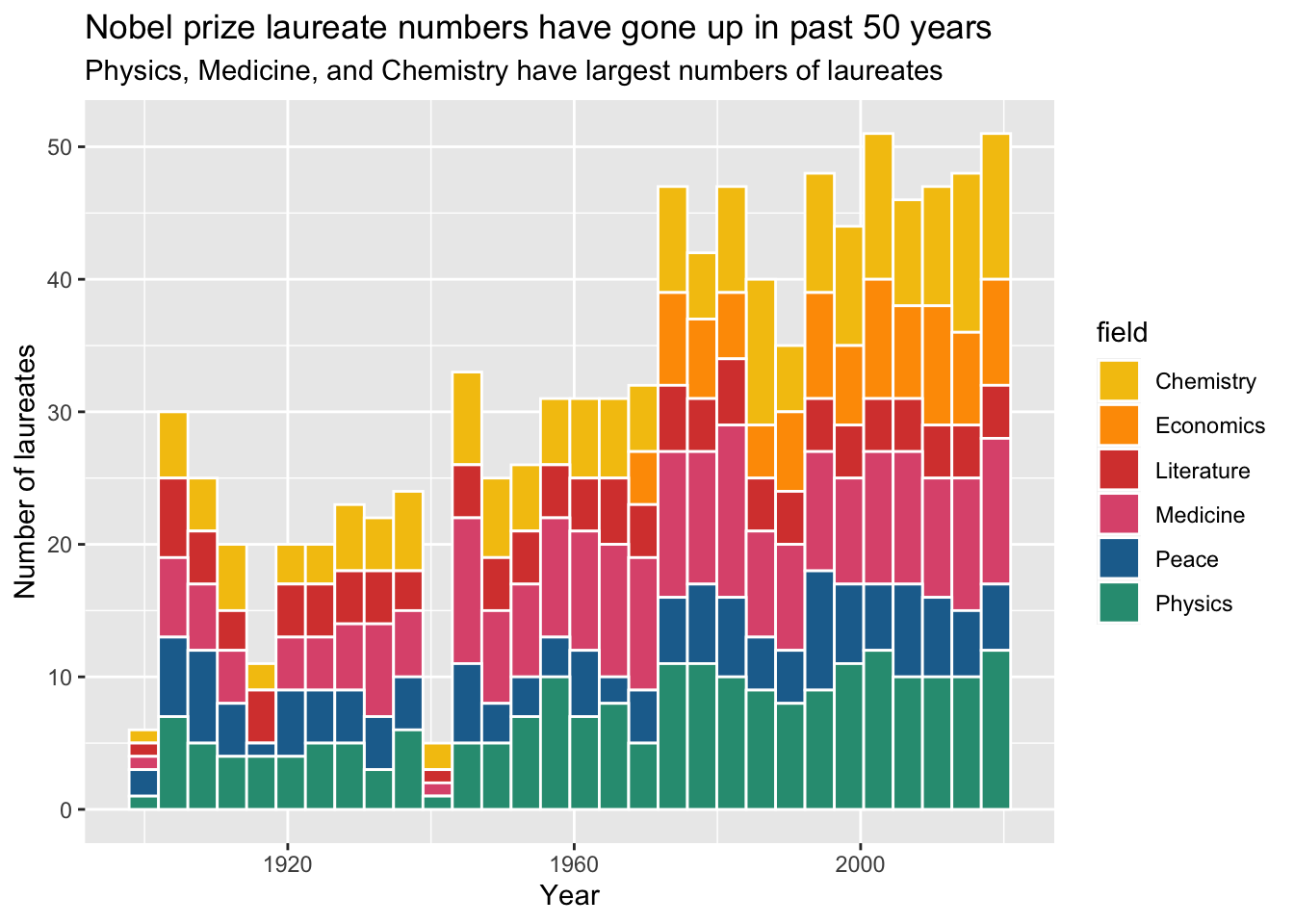Now, how do we get the lowercase “field” to look nicer? When we labeled x and y, they were both in the `aes()`, and it’s the same way with “field”. The fill is in the `aes()`, so we need to have fill in the `labs()`.

Code
``````ggplot(data = nobel,
mapping = aes(x = year,
fill = field)) +
scale_fill_manual(values = met.brewer("Signac", 6)) +
geom_histogram(color = "white") +
labs(title = "Nobel prize laureate numbers have gone up in past 50 years",
subtitle = "Physics, Medicine, and Chemistry have largest numbers of laureates",
x = "Year",
y = "Number of laureates",
fill = "Field")``````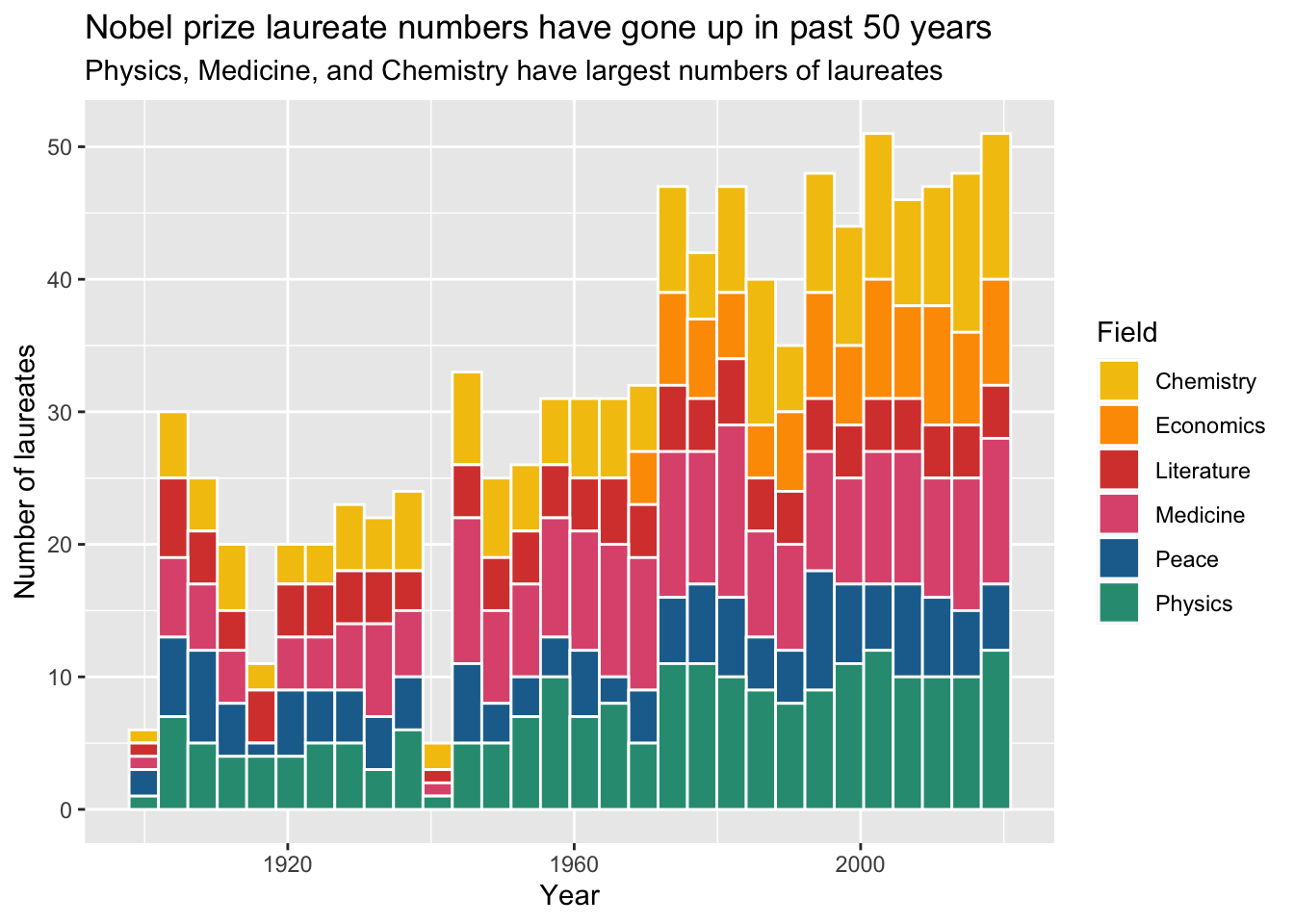Now that is a cool looking graph!